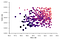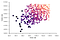# Predicting 800m Times

TL;DR
All times are in milliseconds.
Male Formula:
(1.528 x 400m time) + (0.152 x 1500m time)-1260 = 800m Potential
Female Formula:
(1.419 x 400m time) + (0.213 x 1500m time)-11762 = 800m Potential

The 800 metre event is tough. Ask anyone who has done one. The combination of aerobic and anaerobic systems lead to a very painful two laps if run without caution. Due to it being the meeting point of two energy systems it is harder to traverse to it from the events either side of it - 400m and 1500m - than going from 10k to 5k for example.

There are many different formulas circulating around the track world for predicting an athletes 800m best. There is the classic of “add five seconds to your 400m time and double”. This relationship is something that fascinates me, and although all athletes are different, I want to know how you can predict a sensible 800m time for the average athlete. I also want to know how, if at all, the relationship differs by athlete gender.

# Whats the plan?

I want to create a formula that can predict an athletes potential 800m time, to try and do this I intend to use 400m and/or 1500m bests. The method of going about this is linear regression. I want to know how the formulas from this compare against each other to find an ultimate solution. However in order to do this I will need some data however.

The data being analysed is athletes’ 400m, 800m, and 1500m seasons best for each year from 2013–2015 that is recorded on Power of 10. Power of 10 is a database of British athletic performances for both amateur and professionals. There are 779 different data points: 366 female and 413 male. The data from 2016 is being compared for checking error rates.

# The 400m

The 400m is essentially a sprint, albeit a long one. It makes sense that a faster time would imply in a faster 800m, time but the further the distance the higher proportion of aerobic ability is needed. Consequently an athlete lacking distance work will struggle to match a prediction based on 400m alone.

Despite this there are many different formulas for attempting this. One of the most popular is (400m time + 5 secs) x 2 = 800m time. I will be honest and admit this is one that I often quote, and I believe that it does actually hold up — at least for myself. Is this confirmed by the data?

## Male

As the graph below shows, there is a trend that a faster 400m does equate to a faster 800m. This is appears to be common sense. There are numerous expected outliers and there are many reasons that could cause this. Performing simple linear regression on this results in the formula:

Note: All formulas use times in millisecond. Therefor 54.1 seconds equates to 54100.

(1.8 x 400m time in ms) + 20875 = 800m Potential in ms

The average error for this is 2.5s but how does this compare to the popular formula? That has an average error of 2.8s! It appears that this formula is a minor improvement.

## Female

This is very similar to male, it appears that there is a relationship. The formula produced from this regression is:

(1.98 x 400m time in ms) + 14015 = 800m Potential in ms

The average offset is 3.4s. This is a bigger gap than the male formula, this is possibly due to the higher variation of the women’s time in the data when compared to the male data. The popular formula however has an average error of 4.5s. Again the difference between these is relatively large and shows that the existing methodology is inappropriate.

# The 1500m

Studies have shown that the 800m is around 70% aerobic and as a result of this the second and final lap can be much improved with distance training. It would make sense then that a faster 1500m equates to a faster 800m personal best.

## Men

The trend in the graph below show that the data supports this theory and while there is a large spread, the majority of points fit closely to the regression line. It is no surprise there is a correlation between the two events. A calculation for predicting this relationship is:

(0.197 x 1500m time in ms) + 66439 = 800m Potential

The error on average for this is 2.4s, this edges out the 400m formula slightly but not significantly. A number of other indicators however suggest that this is a favourable predictor.

## Female

Once again the graph shows a correlation between the two events. It is pleasing to see that Laura Muirs’ 2014 season sits almost perfectly on the regression line with times of 2:00.67 and 4:00.07. It will be interesting to calculate this with the formula:

(0.31 x 1500m time in ms) + 46602 = 800m Potential (ms)

Using 4:00.07 (240070 milliseconds) as the 1500m time. The model produces an estimate of 121024 milliseconds — 2:01.023. That is not a bad differential. On average the error is roughly 3.7s, showing that this doesn’t appear to be that bad a model. Although faring slightly worse than the 400m calculator.

# Making a Better Predictor by Using 400m and 1500m

Predicting a potential 800m time can be roughly done using either 400m time or 1500m time. However what would happen if you could combine the two events to create a different calculation? Using the power of multiple linear regression and the magic of data there is an answer!

## MaleGraph of 800m times plotted against 400m/1500m SB. The darker and larger a point, the faster the time.

(1.528 x 400m time) + (0.152 x 1500m time)-1260 = 800m Potential

This formula produces an average differential of 1.9s. This is around .6s better than the previous models. While this may not seem much this is actually around 20% better. There are numerous other methods for calculating the error of this model and comparing it with the previous ones, and in each one of these this combo formula wins substantially producing consistently more accurate predictions.

Let’s take 2016s top British 800m athlete Michael Rimmer and calculate a prediction with him. In 2016 he ran 3:42.3 for 1500m and a 47.9 400m (this was en-route in a 600m, and his only 400m recording of the year). Inputting the numbers produces a calculation of 1:45.69. This is less than a second off his seasons best (1:44.93). In this calculation we are using a 400m that I am sure is undervalued due to being a split of a 600m race. Let’s say that he could have run 47.4, just half a second quicker, in a 400m race. That produces a prediction of 1:44.96. Just 0.03 seconds slower than his time for that season. Pretty good.

## FemaleGraph of 800m times plotted against 400m/1500m SB. The darker and larger a point, the faster the time.

(1.419 x 400m time) + (0.213 x 1500m time)-11762 = 800m Potential

Previous models were only around 3.4s off, whereas this model is on average 2.3s from the actual time. A substantial improvement over previous models, a trend that continues when other ways of quantifying error are used (such as r-squared).

Let’s try an example. Lynsey Sharp sits clear by over a second at the top of 2016s 800m rankings, unfortunately she does not have a 1500m result on Power of Ten from the last few years. Luckily Shelayna Oskan-Clarke has a few 1500m times to utilise. Using her 400m 2016 SB of 53.36 and her 2015 1500m SB of 4:32.52 the model produces a prediction of 2:02.00. This is about 2.5s off of her 2016 SB of 1:59.45. I am not happy with this because in 2014 she recorded a 1500 PB of 4:28.29 which I would say is a fairer representation of her 2016 abilities. So what difference does this make? About .9 seconds worth, predicting a time of 2:01.1. An improvement certainly, and predicting within 2 seconds is credible. On the other hand it makes sense using both 2014 predictor values instead of mixing seasons, this results in a difference of under a second. Not bad.

# Conclusion

Predicting an athletes potential will always result in a rough idea. It is no doubt useful to have a time to aim for however. Dedicated middle distance training will probably see an athlete surpass the predictions calculated here. Nevertheless the predictions should remain a target for the athletes, and being slower than a predicted time could show that there is potentially a weakness that should be worked on.

I hope that splitting the models by gender results in closer predictions. I would be interested to hear about this formula being used in the real world; so please leave some feedback. I hope to revisit this at a later date to delve deeper into the relationship between events and see if there are more insights that can be gained.

Sam Harding

Written by

## More From Medium

### The Doctor for the Undiagnosed

Mar 1, 2019 · 5 min read